# condir: Examples

### Condir

Below we provide two basic examples that use the R package condir. The first example regards data coming from a single group, and the second example data coming from two groups. More details about the package condir are described in the paper by: Krypotos, A. M., Klugkist, I., & Engelhard, I. M. (2017). Bayesian hypothesis testing for human threat conditioning research: An introduction and the condir R package. European Journal of Psychotraumatology, 8.

### Installation

You can install condir via cran using the following command:

install.packages("condir")

For loading condir, use the line below. Please note that for using condir you have to load it at every new R session.

library(condir)

### One group example

Here we show a single group example. Specifically, we first simulate data from a normal distribution for two stimuli, the cs1 and the cs2.

set.seed(1000)
cs1 <- rnorm(50, 5, 5)
cs2 <- rnorm(50, 4.5, 5)

These data correspond to the conditioned responses during the presentation of each stimulus (i.e., the cs1 and the cs2). For comparing the two stimuli, we can use the csCompare function in condir as follow.

tmp <- csCompare(cs1, cs2)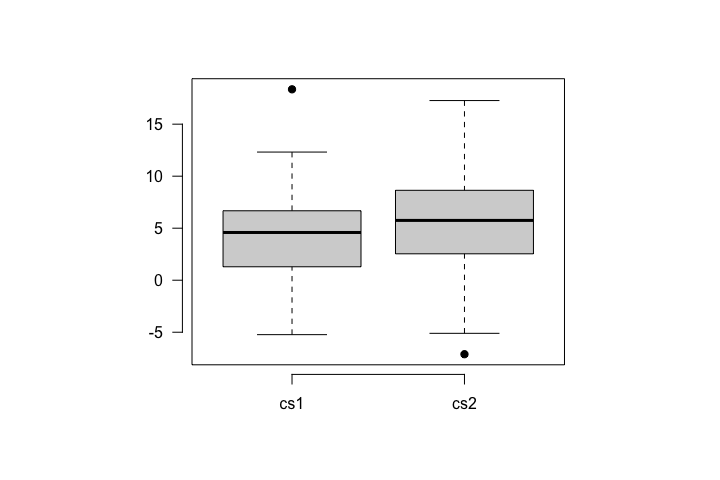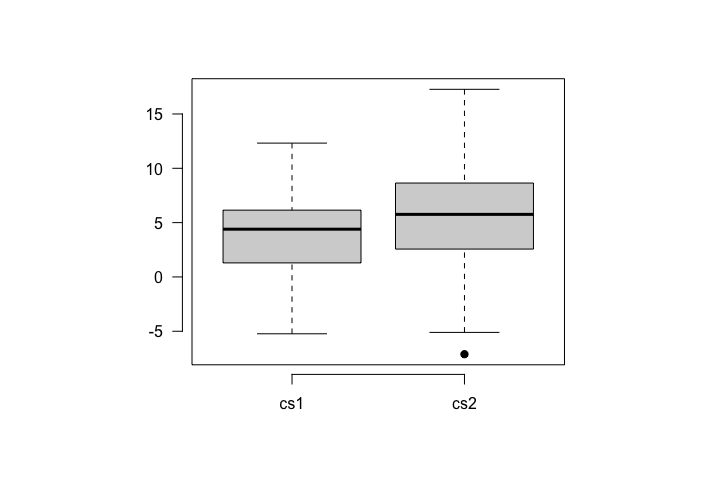tmp
$descriptives vars n mean sd min max range se cs1 1 50 4.21 4.66 -5.23 18.35 23.58 0.66 cs2 2 50 5.46 5.29 -7.11 17.27 24.38 0.75$freq.results
method alternative       WG1       WpG1 WG2 WpG2 null.value       LCI
1 Paired t-test   two.sided 0.9521536 0.04185158   0    0          0 -3.386249
HCI t.statistic df   p.value    cohenD cohenDM    hedgesG hedgesGM
1 0.886061   -1.176018 49 0.2452697 -0.251108   small -0.2472448    small

$bayes.results LNI HNI rscale bf10 bf01 propError 1 -Inf Inf 0.707 0.2944811 3.395803 3.041879e-08$res.out
$descriptives vars n mean sd min max range se cs1 1 49 3.92 4.23 -5.23 12.32 17.55 0.60 cs2 2 49 5.63 5.20 -7.11 17.27 24.38 0.74$freq.results
method alternative       WG1      WpG1 WG2 WpG2 null.value       LCI
1 Paired t-test   two.sided 0.9708112 0.2607728   0    0          0 -3.676637
HCI t.statistic df    p.value     cohenD cohenDM    hedgesG hedgesGM
1 0.2543265   -1.750466 48 0.08642769 -0.3613867   small -0.3557105    small

$bayes.results LNI HNI rscale bf10 bf01 propError 1 -Inf Inf 0.707 0.6383185 1.566616 3.717128e-08 attr(,"class")  "csCompare" attr(,"class")  "csCompare" The data can be plotted with the csPlot function. csPlot(cs1, cs2, ylab = "CRs")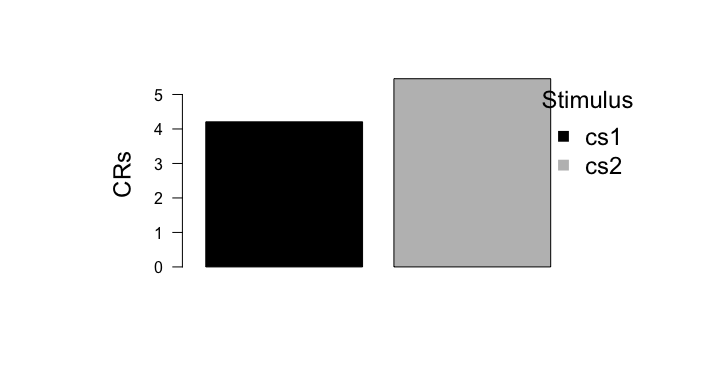In order to make a basic report of the data, we use the csReport function. csReport(tmp) We performed a two sided paired t-test. The results are t (48) = -1.75, p = 0.086, Cohen's d = -0.361 (small effect size). We performed a two sided Bayesian t-test, with a Cauchy prior, with its width set to 0.707. The BF01 was: BF01(0.707) = 1.567. The BF10 was: BF10(0.707) = 0.638.Main analyses We performed a two sided paired t-test. The results are t (49) = -1.176, p = 0.245, Cohen's d = -0.251 (small effect size). We performed a two sided Bayesian t-test, with a Cauchy prior, with its width set to 0.707. The BF01 was: BF01(0.707) = 3.396. The BF10 was: BF10(0.707) = 0.294. **Outliers report** We performed a two sided paired t-test. The results are t (48) = -1.75, p = 0.086, Cohen's d = -0.361 (small effect size). We performed a two sided Bayesian t-test, with a Cauchy prior, with its width set to 0.707. The BF01 was: BF01(0.707) = 1.567. The BF10 was: BF10(0.707) = 0.638. Lastly, the csSensitivity function can be used for a sensitivity analysis, with the csRobustnessPlot function plotting the results. set.seed(1000) tmp <- csSensitivity(cs1, cs2) csRobustnessPlot(cs1, cs2, BF01 = TRUE)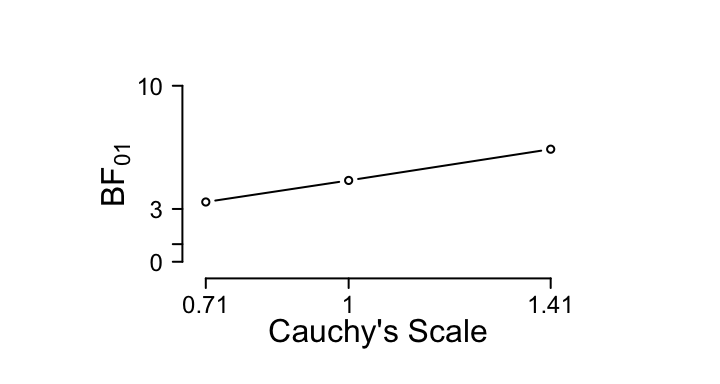The results are now reported with the csReport function. csReport(csSensitivityObj = tmp) We performed a Sensitivity Analysis using the scaling factors: 0.707, 1, 1.41. The results for BF01 were: BF01(0.707) = 1.567, BF01(1) = 2.079, BF01(1.41) = 2.827 respectively. The results for BF10 were: BF10(0.707) = 0.638, BF10(1) = 0.481, BF10(1.41) = 0.354 respectively.Sensitivity analyses We performed a Sensitivity Analysis using the scaling factors: 0.707, 1, 1.41. The results for BF01 were: BF01(0.707) = 3.396, BF01(1) = 4.62, BF01(1.41) = 6.378 respectively. The results for BF10 were: BF10(0.707) = 0.294, BF10(1) = 0.216, BF10(1.41) = 0.157 respectively. **Sensitivity analyses - Outliers report** We performed a Sensitivity Analysis using the scaling factors: 0.707, 1, 1.41. The results for BF01 were: BF01(0.707) = 1.567, BF01(1) = 2.079, BF01(1.41) = 2.827 respectively. The results for BF10 were: BF10(0.707) = 0.638, BF10(1) = 0.481, BF10(1.41) = 0.354 respectively. ### Two groups example The same steps as above are used for the two group example. The only difference is that we now have to define the group allocation by using the group argument – see the first line below. Apart from that, the code is the same as in the example above. That is why we provide the code for this example in a single chunk of code. set.seed(1000) group <- factor(rep(1:2, length(cs1)/2)) tmp <- csCompare(cs1, cs2, group = group)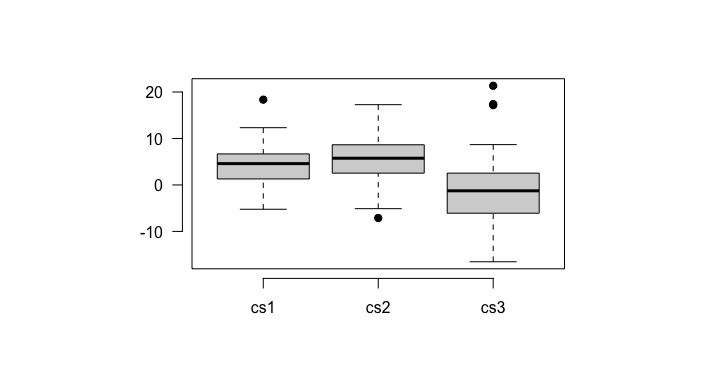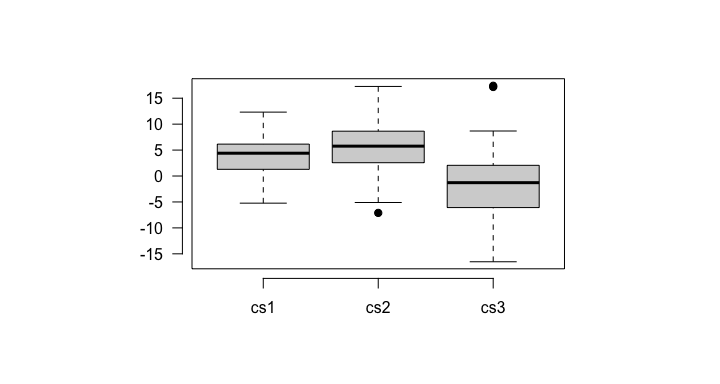tmp $descriptives
$descriptives$1
vars  n  mean   sd    min   max range   se
cs1    1 25  3.07 4.86  -5.23 18.35 23.58 0.97
cs2    2 25  5.16 4.89  -4.45 17.27 21.72 0.98
cs3    3 25 -2.08 6.85 -12.36 21.34 33.70 1.37

$descriptives$2
vars  n  mean   sd    min   max range   se
cs1    1 25  5.34 4.23  -1.87 12.32 14.18 0.85
cs2    2 25  5.76 5.74  -7.11 14.79 21.90 1.15
cs3    3 25 -0.42 8.18 -16.53 17.40 33.93 1.64

$freq.results method alternative WG1 WpG1 WG2 WpG2 1 Welch Two Sample t-test two.sided 0.8810523 0.007265277 0.9687348 0.6131729 null.value LCI HCI t.statistic df p.value cohenD 1 0 -5.961606 2.628604 -0.7807465 46.56861 0.4389025 -0.2509847 cohenDM hedgesG hedgesGM 1 small -0.249059 small$bayes.results
LNI HNI rscale      bf10     bf01    propError
1 -Inf Inf  0.707 0.3630444 2.754484 0.0001990396

$res.out$descriptives
$descriptives$1
vars  n  mean   sd    min   max range   se
cs1    1 24  2.44 3.76  -5.23  9.17 14.40 0.77
cs2    2 24  5.50 4.69  -4.45 17.27 21.72 0.96
cs3    3 24 -3.06 4.91 -12.36  7.07 19.43 1.00

$descriptives$2
vars  n  mean   sd    min   max range   se
cs1    1 25  5.34 4.23  -1.87 12.32 14.18 0.85
cs2    2 25  5.76 5.74  -7.11 14.79 21.90 1.15
cs3    3 25 -0.42 8.18 -16.53 17.40 33.93 1.64

$freq.results method alternative WG1 WpG1 WG2 WpG2 1 Welch Two Sample t-test two.sided 0.9759581 0.8113906 0.9687348 0.6131729 null.value LCI HCI t.statistic df p.value cohenD 1 0 -6.522944 1.237837 -1.376793 39.59676 0.1763113 -0.3612266 cohenDM hedgesG hedgesGM 1 small -0.3583972 small$bayes.results
LNI HNI rscale      bf10    bf01    propError
1 -Inf Inf  0.707 0.6155059 1.62468 6.642139e-05

attr(,"class")
 "csCompare"

attr(,"class")
 "csCompare"
csPlot(cs1, cs2, group = group, ylab = "CRs")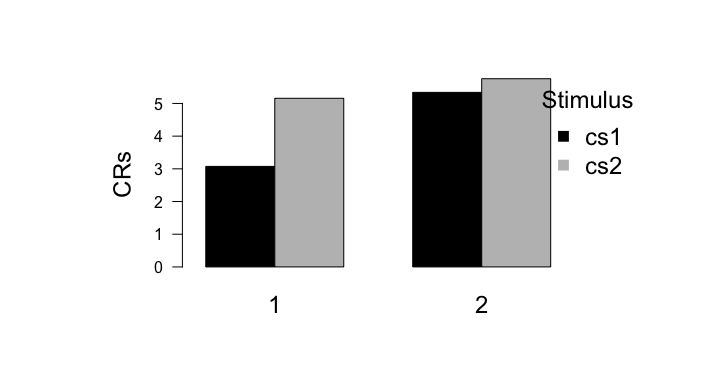csReport(csCompareObj = tmp)
We performed a two sided Welch two sample t-test. The results are t (39.597) = -1.377, p  =  0.176, Cohen's d = -0.361 (small effect size).
We performed a two sided Bayesian t-test, with a Cauchy prior, with its width set to 0.707. The BF01 was: BF01(0.707)  =  1.625. The BF10 was: BF10(0.707)  =  0.616.Main analyses
We performed a two sided Welch two sample t-test. The results are t (46.569) = -0.781, p  =  0.439, Cohen's d = -0.251 (small effect size).
We performed a two sided Bayesian t-test, with a Cauchy prior, with its width set to 0.707. The BF01 was: BF01(0.707)  =  2.754. The BF10 was: BF10(0.707)  =  0.363.

**Outliers report**
We performed a two sided Welch two sample t-test. The results are t (39.597) = -1.377, p  =  0.176, Cohen's d = -0.361 (small effect size).
We performed a two sided Bayesian t-test, with a Cauchy prior, with its width set to 0.707. The BF01 was: BF01(0.707)  =  1.625. The BF10 was: BF10(0.707)  =  0.616.
tmp <- csSensitivity(cs1, cs2)
csRobustnessPlot(cs1, cs2, group, BF01 = TRUE)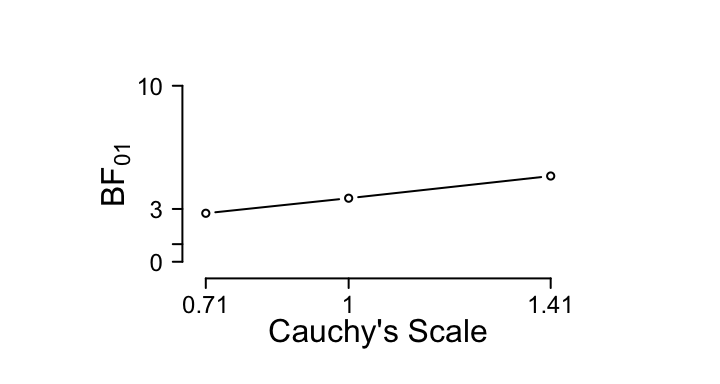csReport(csSensitivityObj = tmp)
We performed a Sensitivity Analysis using the scaling factors: 0.707, 1, 1.41. The results for BF01 were: BF01(0.707)  =  1.567, BF01(1)  =  2.079, BF01(1.41)  =  2.827 respectively. The results for BF10 were: BF10(0.707)  =  0.638, BF10(1)  =  0.481, BF10(1.41)  =  0.354 respectively.Sensitivity analyses
We performed a Sensitivity Analysis using the scaling factors: 0.707, 1, 1.41. The results for BF01 were: BF01(0.707)  =  3.396, BF01(1)  =  4.62, BF01(1.41)  =  6.378 respectively. The results for BF10 were: BF10(0.707)  =  0.294, BF10(1)  =  0.216, BF10(1.41)  =  0.157 respectively.

**Sensitivity analyses - Outliers report**
We performed a Sensitivity Analysis using the scaling factors: 0.707, 1, 1.41. The results for BF01 were: BF01(0.707)  =  1.567, BF01(1)  =  2.079, BF01(1.41)  =  2.827 respectively. The results for BF10 were: BF10(0.707)  =  0.638, BF10(1)  =  0.481, BF10(1.41)  =  0.354 respectively.